# 1715 A Lot More of a Subtraction Distraction

### Today’s Puzzle:

Last time I published a puzzle with the last clue missing. Leaving out the first or the last clue only makes the puzzle slightly more difficult. What if I left out a clue more in the middle of the puzzle. I gave that some thought and designed today’s puzzle. I soon realized that I had to let you know that the 12 is one of the last eight boxes. There is only one solution. Can you find it?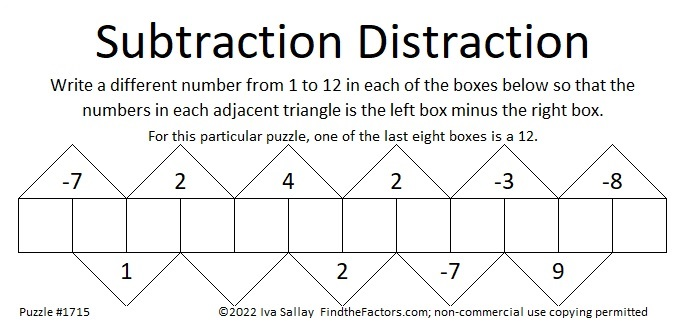I posted a solution video for it on Twitter:

### Factors of 1715:

• 1715 is a composite number.
• Prime factorization: 1715 = 5 × 7 × 7 × 7, which can be written 1715 = 5 × 7³.
• 1715 has at least one exponent greater than 1 in its prime factorization so √1715 can be simplified. Taking the factor pair from the factor pair table below with the largest square number factor, we get √1715 = (√49)(√35) = 7√35.
• The exponents in the prime factorization are 1 and 3. Adding one to each exponent and multiplying we get (1 + 1)(3 + 1) = 2 × 4 = 8. Therefore 1715 has exactly 8 factors.
• The factors of 1715 are outlined with their factor pairs in the graphic below.### More About the number 1715:

1715 = 1·7³·1·5. Thank you, OEIS.org for that fun fact!

1715 is the hypotenuse of a Pythagorean triple:
1029-1372-1715, which is (3-4-5) times 343.

1715 = 5·7³.
5·7º = 2² + 1².
5·7¹ cannot be written as the sum of two squares.
5·7² = 14² + 7².
5·7³ cannot be written as the sum of two squares.
5·7⁴ = 98² + 49².

What do you notice? What do you wonder?

# 1713 A Little More of a Subtraction Distraction

### Today’s Puzzle:

It occurred to me that as long as the last box is neither 1 nor 12 that I could leave the clue above it blank. Can you still solve the puzzle?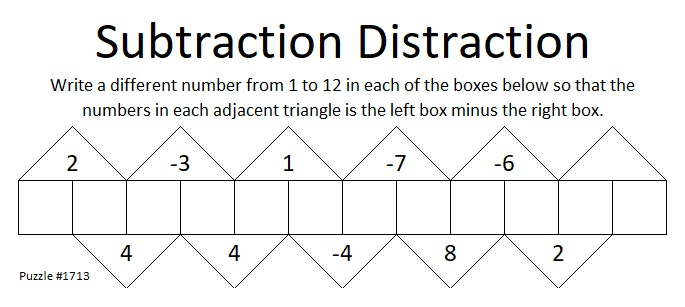### Factors of 1713:

• 1713 is a composite number.
• Prime factorization: 1713 = 3 × 571.
• 1713 has no exponents greater than 1 in its prime factorization, so √1713 cannot be simplified.
• The exponents in the prime factorization are 1 and 1. Adding one to each exponent and multiplying we get (1 + 1)(1 + 1) = 2 × 2 = 4. Therefore 1713 has exactly 4 factors.
• The factors of 1713 are outlined with their factor pair partners in the graphic below.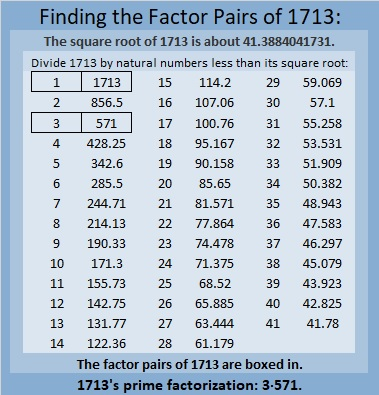### More About the Number 1713:

1713 is the difference of two squares in two different ways:
857² – 856² = 1713, and
287² – 284² = 1713.

1713 is the sum of two, three, and six consecutive numbers:
856 + 857 = 1713,
570 + 571 + 572 = 1713, and
283 + 284 + 285 + 286 + 287 + 288 = 1713.

Do you see any relationship between those two facts?

# 1709 Sometimes “Guess and Check” Is a Good Strategy

### Today’s Puzzle:

Most of the puzzles I publish are logic puzzles, and I encourage you to find the logic of the puzzle and not guess and check.  However, guess and check is a legitimate strategy in mathematics, and it is a legitimate strategy to solve this particular puzzle.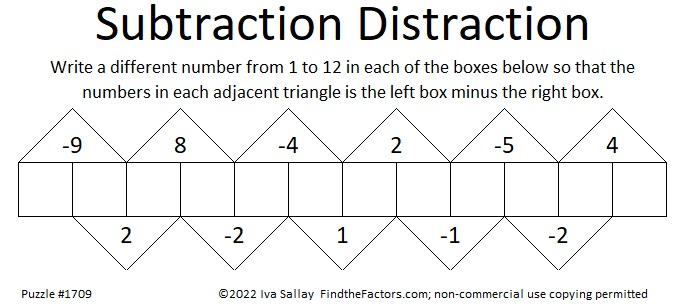Since one of the clues is -9, we know that the two boxes under it must be [1, 10], [2, 11], or [3, 12].

Suppose you assume it’s 1 – 10 = -9. If you fill out the rest of the boxes you would get: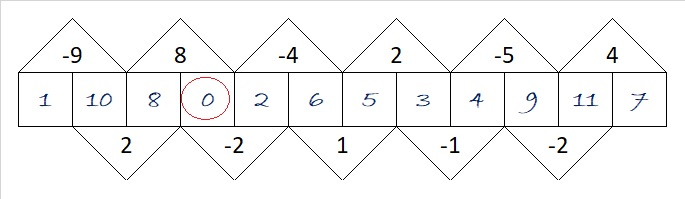You know that isn’t right because zero is not a number from 1 to 12. No problem. Simply add one to each of the numbers you wrote in, and the puzzle will be solved with only numbers from 1 to 12.

Suppose you assumed it’s 3 -12 = -9. The rest of the boxes would look like this:Again, 13 is not included in the numbers from 1 to 12, but you can fix it by subtracting 1 from each of the numbers you wrote in. Easy Peasy.

### Factors of 1709:

• 1709 is a prime number.
• Prime factorization: 1709 is prime.
• 1709 has no exponents greater than 1 in its prime factorization, so √1709 cannot be simplified.
• The exponent in the prime factorization is 1. Adding one to that exponent we get (1 + 1) = 2. Therefore 1709 has exactly 2 factors.
• The factors of 1709 are outlined with their factor pair partners in the graphic below.How do we know that 1709 is a prime number? If 1709 were not a prime number, then it would be divisible by at least one prime number less than or equal to √1709. Since 1709 cannot be divided evenly by 2, 3, 5, 7, 11, 13, 17, 19, 23, 29, 31, 37, or 41, we know that 1709 is a prime number.

### More About the Number 1709:

1709 is the sum of two squares:
35² + 22² = 1709.

1709 is the hypotenuse of a Pythagorean triple:
741-1540-1709, calculated from 35² – 22², 2(35)(22), 35² + 22².

Here’s another way we know that 1709 is a prime number: Since its last two digits divided by 4 leave a remainder of 1, and 35² + 22² = 1709 with 35 and 22 having no common prime factors, 1709 will be prime unless it is divisible by a prime number Pythagorean triple hypotenuse less than or equal to √1709. Since 1709 is not divisible by 5, 13, 17, 29, 37, or 41, we know that 1709 is a prime number.

# 1707 Subtraction Distraction

### Today’s Puzzle:

Even though this Subtraction Distraction puzzle has more boxes than the one I published a couple of weeks ago, it is actually an easier puzzle. Can you write the numbers 1 to 12 in the boxes so that each triangle is its adjacent left box minus its adjacent right box?### Factors of 1707:

1 + 7 + 0 + 7 = 15, a multiple of 3, so 1707 is divisible by 3.

• 1707 is a composite number.
• Prime factorization: 1707 = 3 × 569.
• 1707 has no exponents greater than 1 in its prime factorization, so √1707 cannot be simplified.
• The exponents in the prime factorization are 1 and 1. Adding one to each exponent and multiplying we get (1 + 1)(1 + 1) = 2 × 2 = 4. Therefore 1707 has exactly 4 factors.
• The factors of 1707 are outlined with their factor pair partners in the graphic below.### More About the Number 1707:

1707 is the hypotenuse of a Pythagorean triple:
693-1560-1707, which is 3 times (231-520-569).